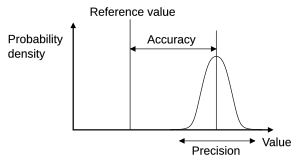# Accuracy and precision facts for kids

Kids Encyclopedia Facts
(Redirected from Accuracy)Accuracy: how close results are to the true value
Precision: the repeatability of measurements

The accuracy and precision of measurements have special meanings in the fields of science, engineering, industry and statistics.

• The accuracy of a measurement system is how close it gets to a quantity's actual (true) value.
• The precision of a measurement system is the degree to which repeated measurements give the same results.

A measurement system can be accurate but not precise, precise but not accurate, neither, or both. For example, if an experiment contains a error in the way it is done, then increasing the sample size generally increases precision but does not improve accuracy. The end result would be a consistent, yet inaccurate, set of results from the flawed experiment. Eliminating the systematic error improves accuracy but does not change precision.

A measurement system is valid if it is both accurate and precise. Related terms include bias (non-random or directed effects caused by a factor or factors unrelated to the independent variable) and error (random variability).

## Related topics

The terminology is also applied to indirect measurements—that is, values obtained by a computational procedure from observed data.

In addition to accuracy and precision, measurements may also have a measurement resolution, which is the smallest change in the underlying physical quantity that produces a response in the measurement.

## Different meanings of 'precision'

The word "precision" also refers to how fine the measurement is made, as the resolution of the measurement, such as to the nearest meter, centimeter, or yard, foot, inch, or nanometer.

In the case of full reproducibility, such as when rounding a number to a representable floating point number, the word precision has a meaning not related to reproducibility. For example, in the IEEE 754-2008 standard, it means the number of bits in the significand (number of digits in the amount), so it is used as a measure for the relative accuracy with which a number can be shown.

## Related pagesAccuracy and precision Facts for Kids. Kiddle Encyclopedia.